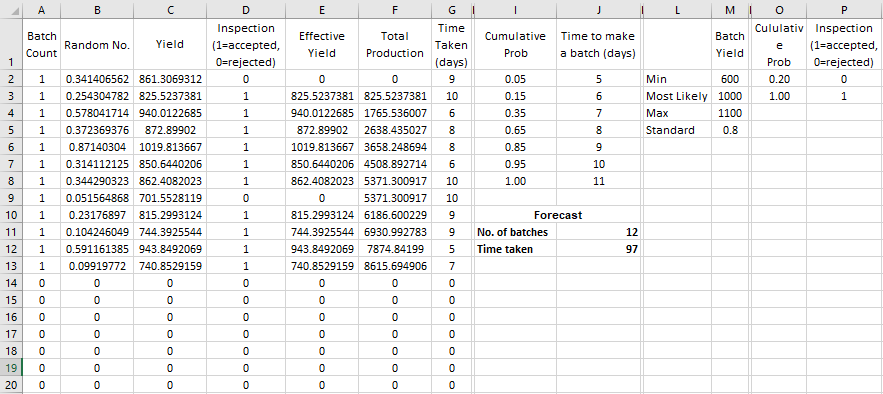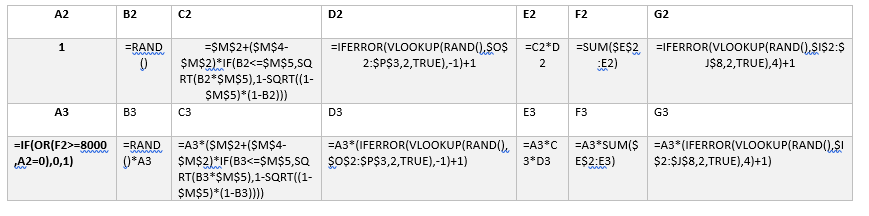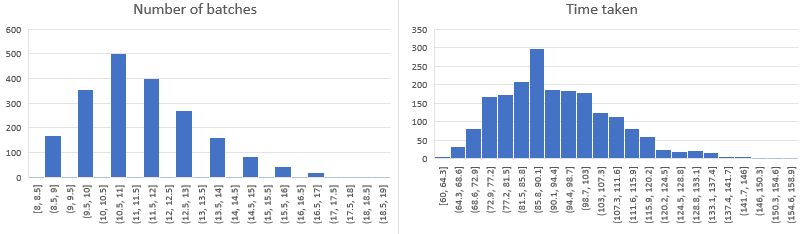# Question & Answer: This question is from Management Science: The Art of Modeling with Spreadsheets…..

This question is from

Management Science: The Art of Modeling with Spreadsheets

Don't use plagiarized sources. Get Your Custom Essay on
Question & Answer: This question is from Management Science: The Art of Modeling with Spreadsheets…..
GET AN ESSAY WRITTEN FOR YOU FROM AS LOW AS \$13/PAGE

Stephen G. Powell and Kenneth R. Baker

John Wiley and Sons 2010 Third Edition

October 15, 2010

Production planning

Your company has agreed to produce 8000 ounces of shampoo for a retailer. This product is manufactured in batches that take 5 to 11 days according to the following distribution (this table is also in the file Shampoo.xlsx).

 Time to make a batch (days) Probability 5 0.05 6 0.10 7 0.20 8 0.30 9 0.20 10 0.10 11 0.05

Only one batch can be made at a time. Each batch yields an uncertain amount of product, ranging between a minimum of 600 ounces and a maximum of 1100 ounces, with a most likely value of 1000 ounces. Finally, 20% of the time a batch fails inspection and the entire batch must be discarded.

Your task is to build a model with which to forecast the number of batches and the number of days that will be required to meet the customer’s order of 8000 ounces.Formula

 A2 B2 C2 D2 E2 F2 G2 1 =RAND() =\$M\$2+(\$M\$4-\$M\$2)*IF(B2<=\$M\$5,SQRT(B2*\$M\$5),1-SQRT((1-\$M\$5)*(1-B2))) =IFERROR(VLOOKUP(RAND(),\$O\$2:\$P\$3,2,TRUE),-1)+1 =C2*D2 =SUM(\$E\$2:E2) =IFERROR(VLOOKUP(RAND(),\$I\$2:\$J\$8,2,TRUE),4)+1 A3 B3 C3 D3 E3 F3 G3 =IF(OR(F2>=8000,A2=0),0,1) =RAND()*A3 =A3*(\$M\$2+(\$M\$4-\$M\$2)*IF(B3<=\$M\$5,SQRT(B3*\$M\$5),1-SQRT((1-\$M\$5)*(1-B3)))) =A3*(IFERROR(VLOOKUP(RAND(),\$O\$2:\$P\$3,2,TRUE),-1)+1) =A3*C3*D3 =A3*SUM(\$E\$2:E3) =A3*(IFERROR(VLOOKUP(RAND(),\$I\$2:\$J\$8,2,TRUE),4)+1)We can simulate the time taken and the number of batches and find the mean value and 95% confidence intervals.

I have simulated them 2000 times and the results and histograms are as following –

 No. of batches Time taken Average 11.71656687 93.36831584 Confidence interval (+/ -) 0.078162631 0.673012787